# GMAT Math : DSQ: Calculating the perimeter of a right triangle

## Example Questions

### Example Question #2655 : Gmat Quantitative Reasoning

What is the perimeter of isosceles triangle ABC?

(1)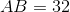(2)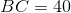Statement 2 ALONE is sufficient to answer the question, but the other statement alone is not sufficient.

EACH statement ALONE is sufficient to answer the question.

Statement 1 ALONE is sufficient to answer the question, but the other statement alone is not sufficient.

BOTH statements TOGETHER are sufficient to answer the question, but NEITHER statement ALONE is sufficient.

BOTH statements TOGETHER are not sufficient to answer the question.

BOTH statements TOGETHER are not sufficient to answer the question.

Explanation:

(1) This statement gives us the length of one side of the triangle. This information is insufficient to solve for the perimeter.

(2) This statement gives us the length of one side of the triangle. This information is insufficient to solve for the perimeter.

Additionally, since we don't know which one of the sides (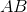or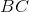) is one of the equal sides, it's impossible to determine the perimeter given the information provided.

### Example Question #181 : Triangles

Find the perimeter of right triangle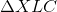.

I)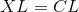II)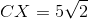Both statements are needed to answer the question.

Statement I is sufficient to answer the question, but statement II is not sufficient to answer the question.

Either statement is sufficient to answer the question.

Statement II is sufficient to answer the question, but statement I is not sufficient to answer the question.

Both statements are needed to answer the question.

Explanation:

If the two shorter sides of a right triangle are equal, that means our other two angles are 45 degrees. This means our triangle follows the ratios for a 45/45/90 triangle, so we can find the remaining sides from the length of the hypotenuse.

I) Tells us we have a 45/45/90 triangle. The ratio of side lengths for a 45/45/90 triangle is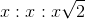.

II) Tells us the length of the hypotenuse.

Together, we can find the remaining two sides and then the perimeter.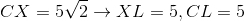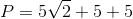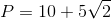### Example Question #2657 : Gmat Quantitative Reasoning

Find the perimeter of the right triangle.

1. The product of the base and height measures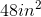.
2. The hypotenuse measures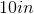.

Statement 2 alone is sufficient, but statement 1 alone is not sufficient to answer the question.

Statements 1 and 2 are not sufficient, and additional data is needed to answer the question.

Each statement alone is sufficient to answer the question.

Statement 1 alone is sufficient, but statement 2 alone is not sufficient to answer the question.

Both statements taken together are sufficient to answer the question, but neither statement alone is sufficient.

Both statements taken together are sufficient to answer the question, but neither statement alone is sufficient.

Explanation:

Statement 1: We need additional information.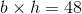But this can mean our base and height measure 2 and 244 and 12, or 6 and 8

We cannot determine which one based solely on this statement.

Statement 2: We're given the length of the hypotenuse so we can narrow down the possible base and height values.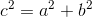We have to see which pair of values makes the statement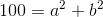true.

The only pair that does is 6 and 8.

We can now find the perimeter of the right triangle: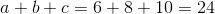or, if you're more familiar with the equation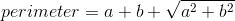, then: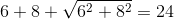### Example Question #2658 : Gmat Quantitative Reasoning

Calculate the perimeter of the triangle.

1. The hypotenuse of the right triangle is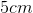.
2. The legs of the right triangle measure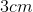and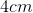Statement 1 alone is sufficient, but statement 2 alone is not sufficient to answer the question.

Each statement alone is sufficient to answer the question.

Statement 2 alone is sufficient, but statement 1 alone is not sufficient to answer the question.

Both statements taken together are sufficient to answer the question, but neither statement alone is sufficient.

Statements 1 and 2 are not sufficient, and additional data is needed to answer the question.

Statement 2 alone is sufficient, but statement 1 alone is not sufficient to answer the question.

Explanation:

Statement 1: In order to find the perimeter of a right triangle, we need to know the lengths of the legs, not the hypotenuse.

Statement 2: Since we have the values to both of the legs' lengths, we can just plug it into the equation for the perimeter: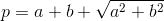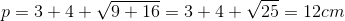Tired of practice problems?

Try live online GMAT prep today.